# All About Throw Keyword in C++

## All About Throw Keyword in C++:

##### Throw Keyword in C++:

The throw keyword in C++ throws an exception when a problem is detected in the code while executing the program. Let us understand the throw keyword in C++ with some examples. Please have a look at the following code.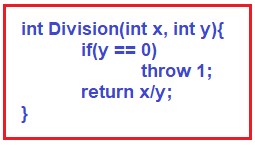We have already seen this example in our previous article. Here, we are throwing 1 means we are throwing an integer value. We want to throw a double value. So, can we throw a double value like the below?
throw 1.5;
Yes, we can throw double value also. We can also throw float value as follows:
throw 5.6f;
If we write ‘f’ then it is a float value otherwise it is a double value.

Can we throw character values like below?
throw ‘a’;
Yes, we can also throw character value. So, the point that you need to remember is we can throw any type of data. We can also throw a string. For example,
throw “Division by 0”;

##### Throwing Custom Exceptions in C++:

As of now, we have seen throwing built-in data types like integer, float, double, string, character, etc. Can we throw a user-defined type using the throw statement in C++? User-defined type is nothing but a class. Suppose we have a class called MyException as follows,
class MyException{
};

So, can we throw this class as follows?
throw MyException;

Yes, we can also throw the object of MyException. For a better understanding, please have a look at the following example. Here, we are throwing the custom MyException object from the Division method using the throw statement in C++.

```#include<iostream>
using namespace std;
#include <exception>

class MyException
{
};

int Division(int a, int b)
{
if (b == 0)
throw MyException();
return a / b;
}

int main()
{
int x = 10, y = 0, z;
try
{
z = Division (x, y);
cout << z << endl;
}
catch (MyException ME)
{
cout << "Division By Zero" << endl;
}
cout << "End of the Program" << endl;
}
```
###### Output: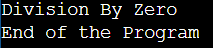##### Inheriting Custom Exception Class from Built-in Exception Class in C++:

If you are throwing your own class exception then better extend your class from the built-in exception class in C++ as follows. But it is not mandatory.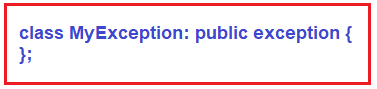So, you can inherit your custom exception class from the exception class in C++. This is not mandatory. If you want then you can inherit from this class. If you are doing this then it is like you are following some hierarchy. For a better understanding, please have a look at the below example.

```#include<iostream>
using namespace std;

class MyException:exception
{
};

int Division(int a, int b)
{
if (b == 0)
throw MyException ();
return a / b;
}

int main()
{
int x = 10, y = 0, z;
try
{
z = Division(x, y);
cout << z << endl;
}
catch(MyException e)
{
cout << "Division By Zero" << endl;
}
cout << "End of the Program" << endl;
}
```
###### Output: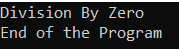##### Overriding the exception class what method in C++:

After inheriting our custom exception class from the built-in exception class, do we have to override anything? Yes, we can override one method which is what method as follows. But this is not mandatory as in our previous example we have not overridden the what method and it is working fine.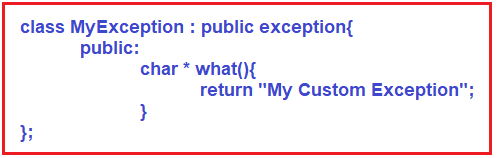So, we can override the what method here. So, if you want then you can override this method, but it is not mandatory. For a better understanding, please have a look at the following example which shows how to override the what method of exception class in C++.

```#include<iostream>
using namespace std;
#include <exception>

class MyException:public exception
{
public:
char *what()
{
return "My Custom Exception";
}
};

int Division(int a, int b)
{
if (b == 0)
throw MyException ();
return a / b;
}

int main()
{
int x = 10, y = 0, z;
try
{
z = Division (x, y);
cout << z << endl;
}
catch (MyException ME)
{
cout << "Division By Zero" << endl;
cout << ME.what () << endl;;
}
cout << "End of the Program" << endl;
}
```
###### Output: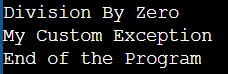Note: what() is a public method provided by the exception class and it has been overridden by all the child exception classes but it is not mandatory. This method returns the cause of an exception.

##### How to make the function throws something in C++?

Now one important point, when a function is throwing, you can declare that this function throws something. For example, please have a look at the below code.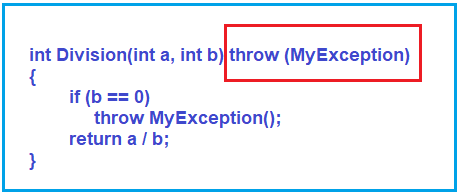So now this Division function declares that it throws some exception i.e. MyException. This is optional in C++. Whether you want to write or not is up to you. If you know about java, there we write throws but here we just write throw. So, if you are throwing an integer value then you can write int in the bracket of a throw like,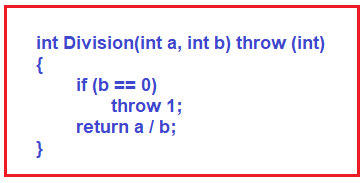So, whatever the type of value you are throwing, you can mention that in the brackets. And if there are more values then you can mention them with commas as follows: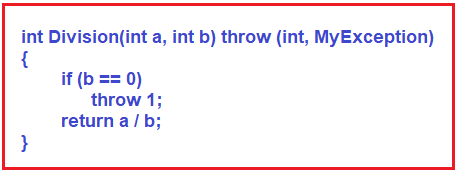Mostly the feature of C++ is such that if you want then do it or don’t want to do then leave it. So, you can make your program better. Suppose other programmers are looking at this function signature then he/she can understand that this function is throwing some exceptions so they should try to catch that exception. If we write empty parenthesis then it means the function doesn’t throw any exception. That’s all about throw keyword in C++.

##### Example to Understand throw Keyword in C++:
```#include<iostream>
using namespace std;
#include <exception>

class MyException:public exception
{
public:
char * what()
{
return "My Custom Exception";
}
};

int Division(int a, int b) throw (int, MyException)
{
if (b == 0)
throw 1;
if (b == 1)
throw MyException();
return a / b;
}

int main()
{
int x = 10, y = 1, z;
try
{
z = Division (x, y);
cout << z << endl;
}
catch (int x)
{
cout << "Division By Zero Error" << endl;
}
catch (MyException ME)
{
cout << "Division By One Error" << endl;
cout << ME.what () << endl;
}
cout << "End of the Program" << endl;
}
```
###### Output: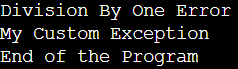##### Can we use cout instead of throw?

If you use cout, the user will know the error. And if you use throw, it will inform the calling function about the error.

##### Are return and throw the same? can return be written in place of throw?

Return is for returning results. The throw is for reporting an error. If you change their roles then the roles of Try and Catch will also change.

##### Can we throw the object of a class instead of the default constructor?

Throwing a constructor will create the object and then throw.

##### Can we throw functions too?

We can throw int, char, double, string, or object of a class.

##### Why class MyException is inheriting from class exception? And in which way it should be inherited (publically, protected, or privately).

For a user-defined exception class, we have to inherit from the exception class provided by C++. We can also override its functionality according to our needs. You can inherit it in any way. It is better to do it using the public.

##### Can we replace try, catch, throw by any name??

try, catch, and throw are keywords. You cannot change them.

##### Can we write else in try block?

Yes, you can write both if-else in the try block.

##### Can we catch an exception for the class object like this?

catch(MyException e)
{
cout<< e << endl;
}

Don’t display it directly, Call what function.
cout<<e->what();

The what() function should return a string message as below :
string what()
{
return “Exception Caught”;
}

##### Why is it necessary to write a catch block for the derived class first and then for the base class?

if we have a base class named “Vehicle” and derived class “Car” for exceptions. if an exception of class “Car” is thrown and the catch block is for “Vehicle” then it will catch the exception of “Car” coz it is also a “Vehicle”. Hence you must always write the catch block of the derived class first. So, if there’s any exception for the derived class, the catch block of the derived class will catch the exception first.